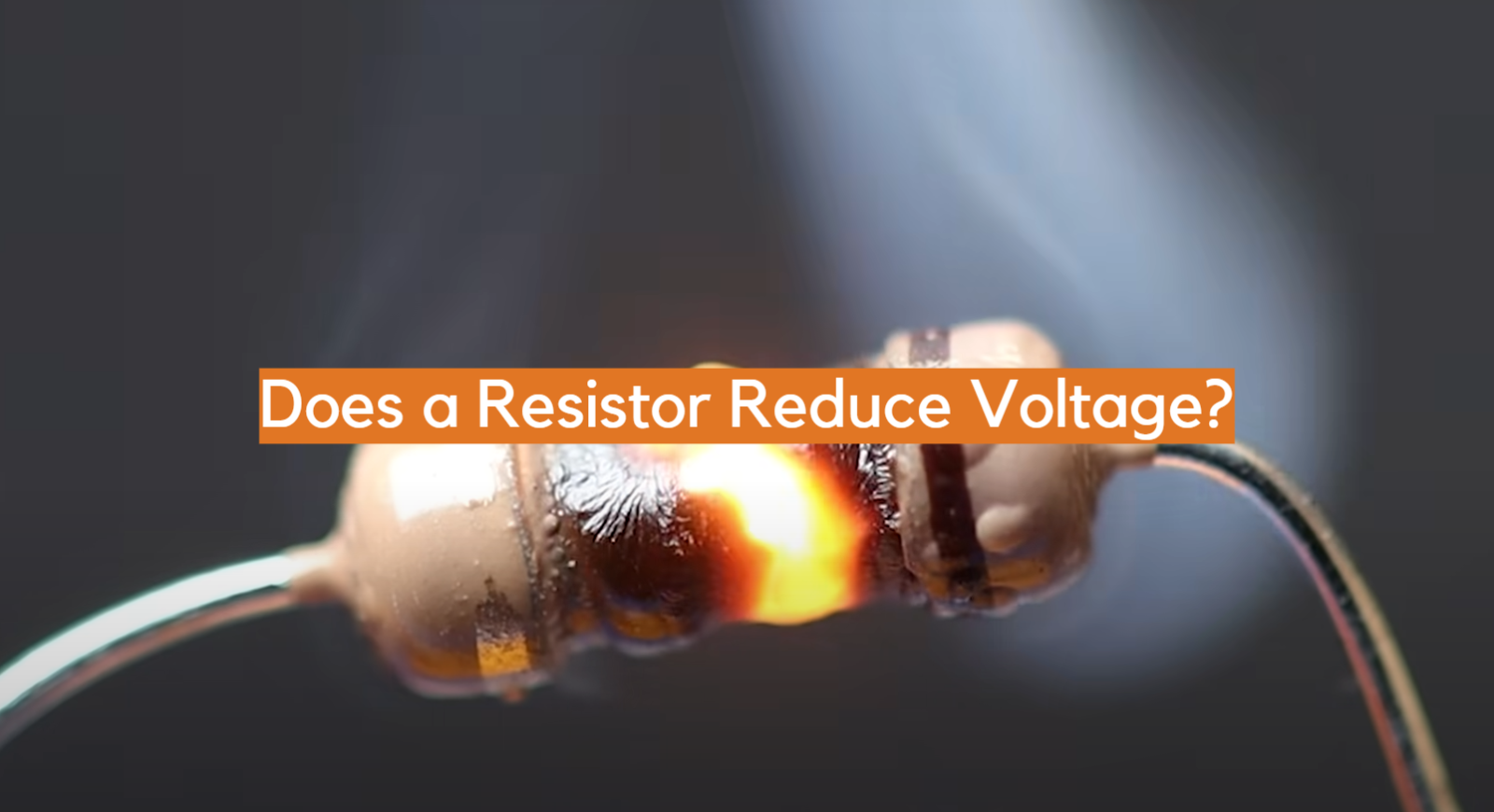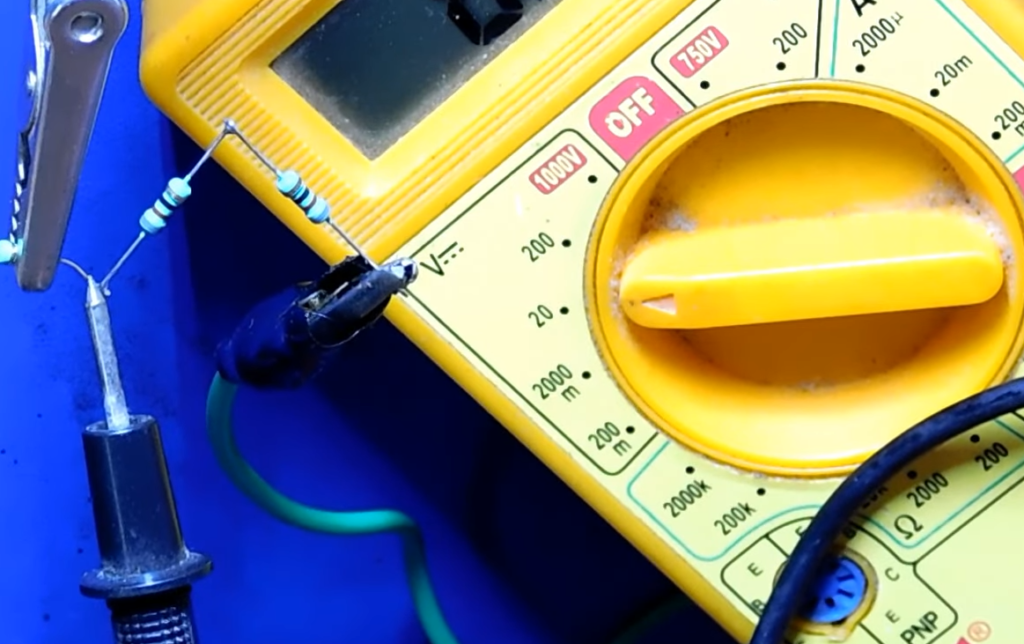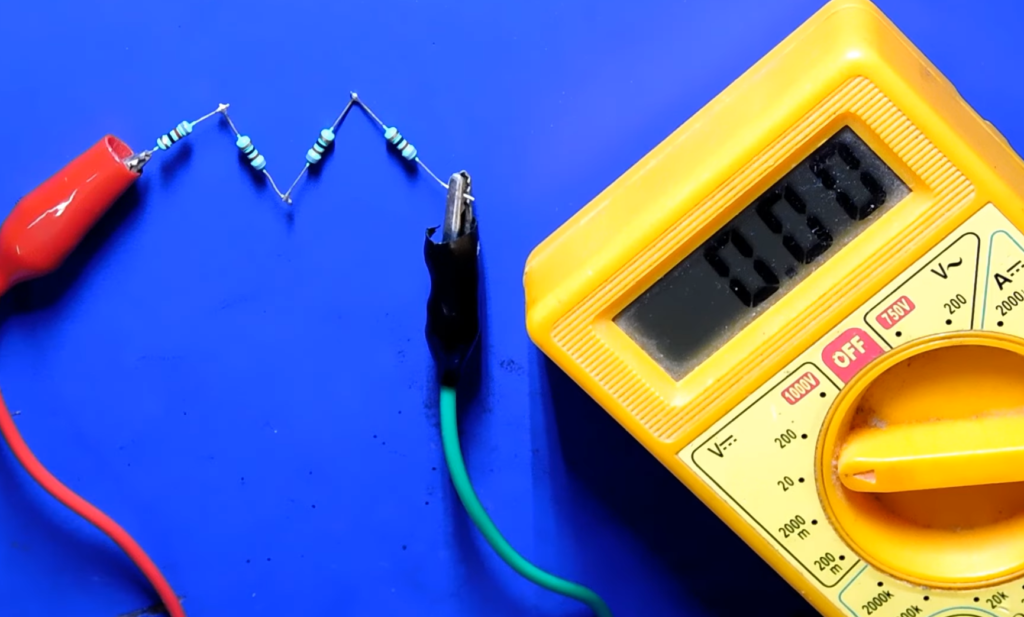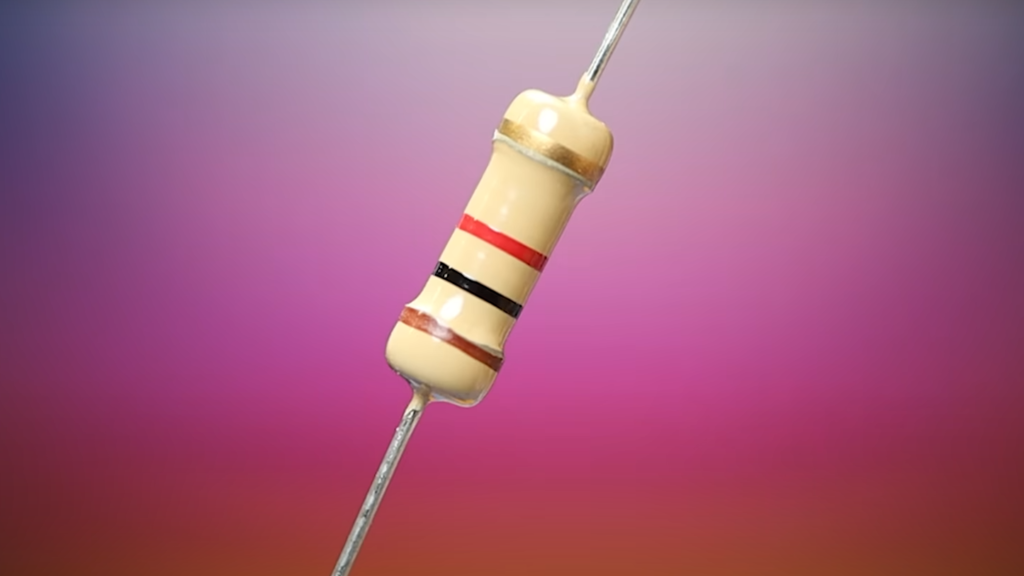# Does a Resistor Reduce Voltage?Resistors are fundamental components in electronic circuits, but there can be confusion about their role in voltage reduction. Understanding the behavior of resistors is crucial for designing and analyzing circuits effectively.

While resistors do not actively reduce voltage like voltage regulators or transformers, they play a vital role in voltage division and controlling current flow.

In this article, we will delve into the concept of voltage reduction in relation to resistors. We will explore how resistors affect voltage, the principles behind voltage drop, and the various techniques used to achieve voltage reduction in practical applications.

By the end, you will have a clear understanding of the role that resistors play in voltage management within electronic circuits.

## What Does A Resistor Do?

### Resistance and Ohm’s Law

A resistor is an electronic component specifically designed to provide resistance to the flow of electrical current. Resistance is measured in ohms (Ω) and determines the opposition encountered by the current in a circuit .According to Ohm’s Law, the current flowing through a conductor is directly proportional to the voltage applied across it and inversely proportional to the resistance. Thus, resistors regulate current flow by controlling the amount of resistance.

### Current Limitation and Protection

One crucial function of resistors is to limit the current in a circuit. By offering a specific resistance, they ensure that the current flowing through other components remains within safe operational limits. This prevents overloading and damage to sensitive electronic devices. For example, in LED circuits, resistors are used to restrict the current to an appropriate level, preventing the LED from burning out due to excessive current.

### Voltage Division

Resistors are also utilized for voltage division purposes. In a series circuit, resistors connected in a sequence divide the total voltage across them in proportion to their respective resistance values. This property is valuable in a variety of applications, such as audio volume control and signal conditioning, where different voltage levels need to be achieved.

### Signal Conditioning

In electronics, resistors play a vital role in signal conditioning. They are used to modify the amplitude, frequency, or shape of electrical signals. By introducing resistance into a circuit, resistors can alter signal levels, match impedance, and attenuate or amplify signals. This capability is particularly useful in audio amplifiers, filters, and equalizers.

### Temperature Sensing and Compensation

Certain types of resistors, such as thermistors and RTDs (Resistance Temperature Detectors), exhibit a change in resistance with temperature variations. These resistors are employed in temperature sensing and compensation circuits. They can accurately measure temperature or compensate for temperature-induced changes in other components, maintaining the stability and reliability of electronic systems.## Important Parameters Of A Resistor:

### Power Rating

The power rating of a resistor indicates the maximum amount of power it can dissipate without overheating. It is usually specified in watts (W). Exceeding the power rating can cause the resistor to fail or even burn. When selecting a resistor, it is crucial to consider the power rating based on the expected current and voltage levels in the circuit.

### Resistance

Resistance is the most fundamental parameter of a resistor. It measures the opposition encountered by the current flow in a circuit and is denoted in ohms (Ω). The resistance value determines the magnitude of current flowing through the resistor, as per Ohm’s Law (V = IR). Resistors with different resistance values are used to achieve desired current levels and voltage drops in electronic circuits.

### Tolerance

Tolerance refers to the permissible variation in the resistance value of a resistor from its nominal or stated value. It is expressed as a percentage. For instance, a resistor with a 10% tolerance could have a resistance that deviates by up to 10% from the specified value. Tolerance ensures that resistors are manufactured within acceptable limits and aids in achieving accurate circuit designs.

### Temperature Coefficient

The temperature coefficient of a resistor indicates the change in resistance with temperature variations. Different resistor materials exhibit different temperature coefficients, represented by a coefficient value in parts per million per degree Celsius (ppm/°C). It is essential to consider the temperature coefficient when using resistors in applications where temperature fluctuations may affect their performance or accuracy.

### Capacitance and Inductance

While resistors primarily provide resistance, they can also possess parasitic capacitance and inductance. These properties arise due to the physical construction and materials used in the resistor. Capacitance and inductance in resistors are typically small and insignificant for most applications. However, in high-frequency circuits or applications where precise impedance matching is crucial, these parameters become relevant and may require consideration .## The Resistor Reducing Voltage Guide:

### Does a Resistor Reduce Voltage or Current?

To clarify the confusion, a resistor primarily reduces voltage. It achieves this by introducing resistance into a circuit, causing a voltage drop across itself. However, it is important to note that this voltage drop indirectly affects the current flow through the resistor, as per Ohm’s Law.

### What is Voltage Drop Across a Resistor?

Voltage drop refers to the difference in voltage across a component or element in an electrical circuit. When current flows through a resistor, there is a voltage drop across it due to the resistance it provides. The magnitude of the voltage drop depends on the current passing through the resistor and its resistance value.

### Why Does Voltage Drop Occur Across a Resistor?

Voltage drop occurs across a resistor due to the resistance it offers to the flow of electric current. As current passes through the resistor, some of the electrical energy is converted into heat, resulting in a decrease in voltage across the component.

### Does Voltage Drop Occur Across a Single Resistor?

Voltage drop can occur across a single resistor when it is connected in a circuit. If a resistor is the only component connected between two points with a potential difference, then the entire voltage across those points will be dropped across the resistor.

### How Much Voltage Drops Across a Resistor?

The voltage drop across a resistor can be calculated using Ohm’s Law: V = IR. The voltage drop (V) is equal to the product of the current (I) flowing through the resistor and its resistance (R).

### Why Is There a Voltage Drop Across a Resistor?

Voltage drop occurs across a resistor due to the conversion of electrical energy into heat. The resistor restricts the flow of current, and as electrons pass through it, they collide with atoms in the resistor material, generating heat and resulting in a voltage drop.

### Why Do You Need a Resistor to Reduce Current?

Resistors are commonly used to reduce current in circuits. By introducing resistance, they limit the amount of current flowing through a circuit, preventing damage to sensitive components and ensuring safe operation.

### Why Would You Use a Resistor to Reduce Voltage?

Resistors can also be used to reduce voltage in certain applications. By connecting a resistor in series with a load or component, the voltage across the load can be lowered. This technique is commonly employed in voltage dividers and to control signal levels.### What Are the Different Types of Resistors Used to Reduce Current and Voltage?

Various types of resistors can be used to reduce current and voltage, including carbon composition resistors, metal film resistors, wirewound resistors, and variable resistors (potentiometers). Each type offers different characteristics suitable for specific applications.

### How Does a Resistor Reduce Current?

A resistor reduces current by offering resistance in a circuit, which limits the flow of electrons. The resistance value determines the extent to which the current is reduced. This property is utilized in current-limiting applications and to protect components from excessive current flow.

### How is a Resistor Constructed to Reduce Current?

Resistors are constructed using materials with high resistivity, such as carbon, metal films, or wire wound around a core. The length, thickness, and composition of the resistor material determine its resistance value. By varying these parameters during construction, resistors with different resistance values can be manufactured to suit specific current-reducing requirements.

## How A Resistor Can Reduce Voltage:

### Voltage Drop Across Its Terminals

When a resistor is connected in a circuit, a voltage drop occurs across its terminals due to the resistance it offers. This voltage drop is a result of the conversion of electrical energy into heat as current passes through the resistor. The magnitude of the voltage drop depends on the current flowing through the resistor and its resistance value, as described by Ohm’s Law (V = IR).

### Voltage Divider

Another way a resistor can reduce voltage is by being part of a voltage divider circuit. A voltage divider is a network of resistors connected in series or in series-parallel configuration. By strategically selecting the resistance values, the voltage across a specific resistor in the divider can be reduced. The reduced voltage can then be utilized for various applications in the circuit.

In a voltage divider, the output voltage is determined by the ratio of the resistances involved.According to the voltage divider equation, the output voltage (Vout) is equal to the input voltage (Vin) multiplied by the ratio of the second resistor’s resistance (R2) to the sum of both resistances (R1 + R2) :

Vout = Vin * (R2 / (R1 + R2))

By varying the resistance values, the output voltage can be adjusted to a desired level. This property is commonly employed in various electronic circuits, such as level shifting, signal conditioning, and biasing.

The voltage divider configuration is widely used in potentiometers or variable resistors. By adjusting the position of the wiper on the resistor element, the resistance ratio changes, resulting in a variable output voltage. Potentiometers are often used for volume control, brightness adjustment, and other applications where variable voltage levels are required.

## How to Reduce Voltage with Resistors:

### Reducing Voltage in Half

To reduce voltage in half using resistors, you can utilize a voltage divider circuit. A voltage divider consists of two resistors connected in series, with the output voltage taken from the junction between the resistors.

To achieve a voltage reduction of 50%, you need to ensure that the two resistors have the same resistance value.

Let’s assume you have an input voltage (Vin) that you want to reduce by half. By selecting two resistors of equal resistance, the output voltage (Vout) can be calculated using the voltage divider equation :

Vout = Vin * (R2 / (R1 + R2))

To achieve a voltage reduction in half, set R1 = R2. For example, if the input voltage is 10V, you can choose two resistors of equal resistance, such as 10kΩ each. The resulting output voltage will be 5V.### Reducing Voltage to Any Value

If you need to reduce voltage to a specific value that is not necessarily half of the input voltage, you can still employ a voltage divider circuit. In this case, you will need to choose different resistance values for the two resistors.

To calculate the required resistance values, you can rearrange the voltage divider equation as follows:

R2 = (Vout / Vin) * R1

Here, Vout is the desired output voltage, Vin is the input voltage, and R1 is the resistance connected to the input voltage. By selecting a value for R1, you can calculate the resistance value for R2 to achieve the desired output voltage.

For example, if you have an input voltage of 12V and you want to reduce it to 6V, you can choose a value for R1, such as 10kΩ. Plugging the values into the equation, you can calculate R2 :

R2 = (6V / 12V) * 10kΩ = 5kΩ

By using a 10kΩ resistor for R1 and a 5kΩ resistor for R2, you can achieve a voltage reduction from 12V to 6V.## FAQ:

### 1. How to reduce voltage from 12V to 5V using a resistor?

To reduce voltage from 12V to 5V using a resistor, you can employ a voltage divider circuit. The required resistor values can be calculated using the voltage divider equation. Let’s assume you want to reduce the voltage from 12V to 5V.

You can choose a resistor (R1) to be connected to the input voltage (12V) and calculate the resistance value for the second resistor (R2) using the equation:

R2 = (Vout / Vin) * R1

Plugging in the values, R2 = (5V / 12V) * R1. By selecting a value for R1, you can calculate the corresponding resistance for R2.

For instance, if you choose R1 = 10kΩ, then R2 would be approximately 4.17kΩ. Connecting these resistors in series between the 12V source and ground will result in a voltage drop across R2, reducing the voltage to approximately 5V .

### 2. How to convert a 12V DC to 6V DC using a resistor?

It is not possible to directly convert a 12V DC voltage to 6V DC using just a resistor. Resistors are passive components that only provide resistance and cannot actively change or convert voltages. To achieve a step-down conversion from 12V to 6V DC, you would need to use a voltage regulator or a buck converter circuit .

### 3. Can you reduce voltage from 24V to 5V using a resistor?

It is not practical to reduce voltage from 24V to 5V using only a resistor. The voltage drop across a resistor is determined by the current flowing through it and its resistance value (V = IR).

A resistor alone cannot provide the necessary voltage reduction from 24V to 5V, as it would require an extremely high resistance value, resulting in significant power dissipation and heat generation.

To achieve such voltage conversion, you would need to use a voltage regulator or a step-down converter circuit.

### 4. Can you reduce voltage from 12V to 3V using a resistor?

Similar to the previous question, it is not feasible to reduce voltage from 12V to 3V using just a resistor. The voltage drop across a resistor is determined by the current flowing through it and its resistance value.

Achieving a voltage reduction from 12V to 3V using a resistor alone would require an impractically high resistance value and result in significant power dissipation. To achieve this voltage conversion, a voltage regulator or a step-down converter circuit would be necessary.

### 5. How many resistors are required to reduce the voltage from 12V to 9V?

To reduce the voltage from 12V to 9V using resistors, you can employ a voltage divider circuit. Since the voltage divider equation requires two resistors, you would need two resistors in this case. By selecting appropriate resistance values, you can determine the voltage division ratio that achieves the desired output voltage of 9V.

### 6. Does voltage decrease across a resistor?

Yes, voltage decreases across a resistor. When current flows through a resistor, the resistor introduces resistance, which converts electrical energy into heat. This conversion results in a voltage drop across the resistor, according to Ohm’s Law (V = IR).

### 7. Does a resistor reduce power?

Yes, a resistor reduces power. When current flows through a resistor, power is dissipated in the form of heat due to the resistance. The power dissipated in a resistor can be calculated using the formula P = IV, where P is power, I is current, and V is voltage. The power dissipation in a resistor causes a reduction in the overall power in the circuit.

### 8. Does adding a resistor decrease voltage or current?

Adding a resistor in a circuit can affect both voltage and current. The voltage across a resistor depends on the current flowing through it and its resistance value. By increasing the resistance, the voltage drop across the resistor increases, resulting in a decrease in the voltage downstream from the resistor.

Additionally, according to Ohm’s Law, when resistance increases, current decreases, and vice versa. Therefore, adding a resistor can decrease both voltage and current in a circuit.

### 9. Why does voltage drop after a resistor?

Voltage drops after a resistor because the resistor introduces resistance to the flow of current. As current passes through the resistor, some of its energy is converted into heat due to the resistance. This conversion of electrical energy into heat results in a voltage drop across the resistor. The magnitude of the voltage drop is proportional to the current flowing through the resistor and its resistance value, as given by Ohm’s Law (V = IR).

### 10. Does voltage stay the same after a resistor?

No, voltage does not stay the same after a resistor. As current flows through a resistor, the resistor introduces resistance and converts electrical energy into heat. This conversion leads to a voltage drop across the resistor. The magnitude of the voltage drop depends on the current flowing through the resistor and its resistance value. Therefore, the voltage decreases after a resistor.

### 11. Does high resistance cause voltage drop?

Yes, high resistance causes a higher voltage drop. According to Ohm’s Law (V = IR), the voltage drop across a resistor is directly proportional to its resistance. Therefore, a higher resistance value will result in a larger voltage drop for the same current flowing through the resistor.

### 12. How do you reduce voltage drop?

To reduce voltage drop, several strategies can be employed:

• Use thicker wires or cables with lower resistance to minimize the resistance along the path;
• Reduce the length of the wire or cable to decrease the overall resistance;
• Increase the conductor’s cross-sectional area to reduce resistance;
• Utilize voltage regulators or power conditioning devices to stabilize and regulate voltage levels in the circuit;
• Minimize the number of connections and junctions, as they can introduce additional resistance and contribute to voltage drop;

### 13. What prevents voltage drop?

To prevent voltage drop, you can take several measures:

• Use thicker wires or cables with lower resistance to minimize resistance and voltage drop;
• Keep the length of the wires or cables as short as possible to reduce the overall resistance;
• Choose conductors with a larger cross-sectional area to decrease resistance;
• Opt for higher voltage levels in power distribution to minimize the impact of voltage drop over long distances;
• Utilize voltage regulators or compensating devices to maintain stable voltage levels throughout the circuit;

### 14. What happens if the voltage is too low?

If the voltage is too low, it can adversely affect the performance of electrical and electronic devices. Insufficient voltage may result in inadequate power supply, causing devices to malfunction, operate at reduced efficiency, or fail to function altogether.

Lower voltages can lead to dim lighting, slower motor speeds, and inaccurate sensor readings, among other issues. It is important to ensure that devices receive the required voltage levels to operate optimally and reliably.

### 15. How do you reduce 5V to 3.3V with a resistor?

Reducing 5V to 3.3V using only a resistor is not practical, as resistors alone cannot actively regulate or convert voltage levels. To achieve this voltage reduction, you would need to use voltage regulators or voltage divider circuits designed specifically for this purpose. These components can provide stable and precise voltage reduction from 5V to 3.3V.

### 16. What size resistor for 12V to 5V?

To determine the appropriate resistor size for reducing voltage from 12V to 5V, you would need to consider the desired current flowing through the circuit and the specific voltage drop requirements.

Using Ohm’s Law (R = V/I), you can calculate the required resistance value based on the current and voltage drop across the resistor. Additionally, it is important to choose a resistor with a power rating suitable for the anticipated power dissipation to prevent overheating and potential damage.

### 17. How to reduce 220V to 5V?

Reducing voltage from 220V to 5V requires more than just a resistor, as the voltage drop across a resistor alone cannot achieve such a significant reduction. To convert 220V AC voltage to 5V DC voltage, you would need to use a step-down transformer followed by a rectifier and voltage regulator circuit. The transformer reduces the voltage to a suitable level, and the rectifier converts the AC voltage to DC. The voltage regulator then ensures a stable output of 5V .

### 18. Do resistors increase current?

No, resistors do not increase current. In fact, resistors impede the flow of current by introducing resistance. According to Ohm’s Law (I = V/R), current is inversely proportional to resistance. When resistance increases, current decreases, and when resistance decreases, current increases. Therefore, resistors limit or decrease the current flowing through them.

### 19. What does a resistor do?

A resistor is an electronic component that provides resistance to the flow of electrical current in a circuit. It restricts the flow of current, converting electrical energy into heat. Resistors are commonly used to control current levels, divide voltage, limit current flow, set biasing conditions, and stabilize circuits, among many other applications.

### 20. How do you reduce voltage in a circuit?

To reduce voltage in a circuit, you can use voltage dividers, voltage regulators, or step-down transformers. Voltage dividers utilize resistors to create a fraction of the input voltage at the output.

Voltage regulators are active components that maintain a constant output voltage regardless of input variations. Step-down transformers reduce voltage levels in AC circuits by changing the turns ratio between the primary and secondary windings.

### 21. Are resistors 100% efficient?

Resistors themselves are passive components and do not consume any power. However, they dissipate power in the form of heat due to the electrical energy converted by the resistance. Therefore, resistors are not 100% efficient. Some power is lost as heat during the process, resulting in less electrical energy being utilized for the intended purpose.

The efficiency of a resistor depends on its construction and the materials used, but it is typically high, with most of the electrical energy being dissipated as heat.